# White and black dot detection using OpenCV Python

OpenCV python is one of the image processing libraries in python and it uses the Numpy array to store the image data so that all the image arrays are represented as a ndarray type.

The cv2.findContours() method in the python OpenCV module is used for detecting the objects in a binary image. In the article below, we will use this method to detect the White and Black dots (objects) in an image. Following is the syntax of this method –

cv2.findContours(image, mode, method[, contours[, hierarchy[, offset]]])


Where,

• Image is an 8-bit single-channel image (binary image).

• Contours is the detected contours.

### Approach

We will follow the below steps to detect/count black and white dots in an image using the OpenCV module.

• Convert the image to grayscale.

• Apply median blur to smoothen the image.

• Define the Thresholds.

• Find contours

• Iterate through contours and filter using the contour area

## Using the cv2.findContours() method

Along with the cv2.findContours() method we will use the below methods also −

• cv2.medianBlur(): to smoothen the input image.

• cv2.cvtColor(): to convert the color image to a grayscale image.

• cv2.threshold(): to define the Thresholds.

• cv2.contourArea(): filter the contours (dots) based on the area

### Example

Let’s take an input image "WhiteDots2.jpg'” to detect the white dots on a dark background image.

import cv2

blur = cv2.medianBlur(image, 5)
gray = cv2.cvtColor(blur, cv2.COLOR_BGR2GRAY)
thresh = cv2.threshold(gray,200,255, cv2.THRESH_BINARY)

cnts = cv2.findContours(thresh, cv2.RETR_EXTERNAL, cv2.CHAIN_APPROX_SIMPLE)
cnts = cnts if len(cnts) == 2 else cnts

min_area = 0.1
white_dots = []
for c in cnts:
area = cv2.contourArea(c)
if area > min_area:
cv2.drawContours(image, [c], -1, (36, 255, 12), 2)
white_dots.append(c)

print("White Dots count is:",len(white_dots))
cv2.imshow('image', image)
cv2.waitKey()


### Output

White Dots count is: 11


### Input Image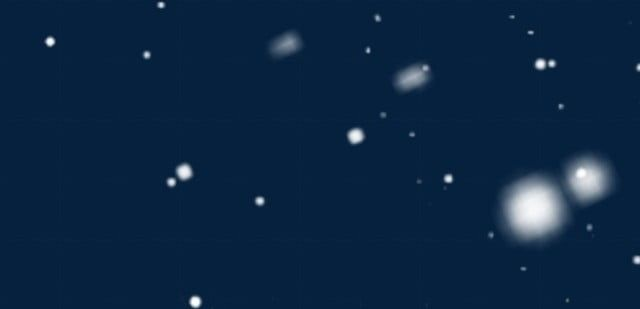### Output Image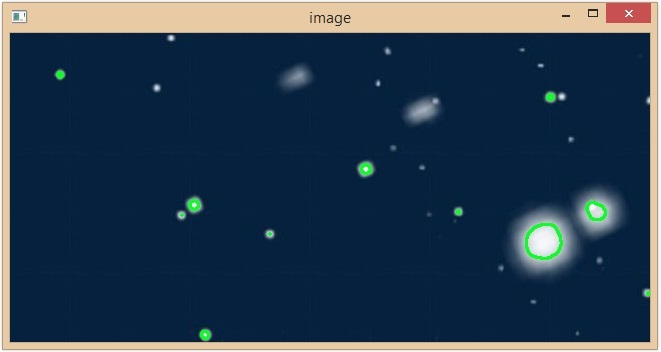In the above example, we have successfully detected 16 white dots on the input image and all are highlighted with a green color.

### Example

In this example, we will take an input image "black-doted-butterflies.jpg'” to detect the black dots on a white background image.

import cv2

blur = cv2.medianBlur(img, 5)
gray = cv2.cvtColor(blur, cv2.COLOR_BGR2GRAY)
thresh = cv2.threshold(gray,100,255, cv2.THRESH_BINARY_INV)

cnts = cv2.findContours(thresh, cv2.RETR_EXTERNAL, cv2.CHAIN_APPROX_SIMPLE)
cnts = cnts if len(cnts) == 2 else cnts

min_area = 10
black_dots = []
for c in cnts:
area = cv2.contourArea(c)
if area > min_area:
cv2.drawContours(img, [c], -1, (36, 255, 12), 2)
black_dots.append(c)

print("Black Dots Count is:",len(black_dots))
cv2.imshow('Output image', img)
cv2.waitKey()


### Output

Black Dots Count is: 25


### Input Image### Output Image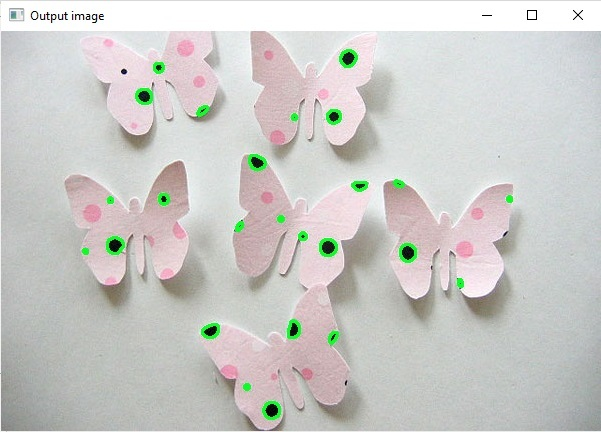By using the cv2.findContours() method we have successfully detected 25 black dots on the butterflies image. The detected black dots are highlighted with a green color.

### Example

In this example, we will take an input image "BlackAndWhite.jpg” to detect both black and white dots in a single image.

import cv2

blur = cv2.medianBlur(img, 5)
gray = cv2.cvtColor(blur, cv2.COLOR_BGR2GRAY)

thresh_for_black_dots = cv2.threshold(gray,100,255, cv2.THRESH_BINARY_INV)
thresh_for_white_dots = cv2.threshold(gray,200,255, cv2.THRESH_BINARY)

cnts_for_black_dots = cv2.findContours(thresh_for_black_dots, cv2.RETR_EXTERNAL, cv2.CHAIN_APPROX_SIMPLE)
cnts_for_white_dots = cv2.findContours(thresh_for_white_dots, cv2.RETR_EXTERNAL, cv2.CHAIN_APPROX_SIMPLE)

cnts_for_black_dots = cnts_for_black_dots if len(cnts_for_black_dots) == 2 else cnts_for_black_dots
cnts_for_white_dots = cnts_for_white_dots if len(cnts_for_white_dots) == 2 else cnts_for_white_dots

min_area = 1
black_dots = []
white_dots = []

for c in cnts_for_white_dots:
area = cv2.contourArea(c)
if area > min_area:
cv2.drawContours(img, [c], -1, (36, 255, 12), 2)
black_dots.append(c)
white_dots.append(c)

for c in cnts_for_black_dots:
area = cv2.contourArea(c)
if area > min_area:
cv2.drawContours(img, [c], -1, (36, 255, 12), 2)
black_dots.append(c)

print("Black Dots Count is:",len(black_dots))
print("White Dots count is:",len(white_dots))

cv2.imshow('Output image:', img)
cv2.waitKey()


### Output

Black Dots Count is: 249
White Dots count is: 134


We have successfully detected the black and white dots using the python OpenCV library in different approaches.

### Input Image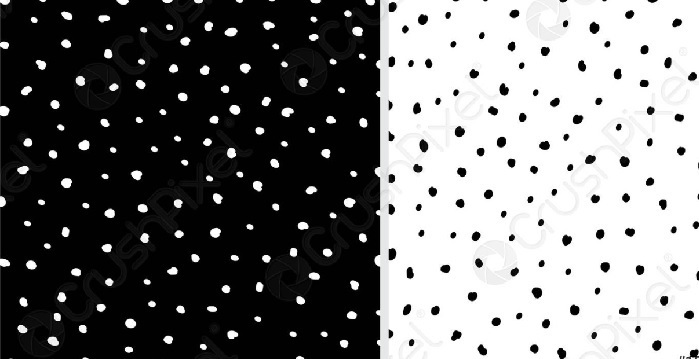### Output Image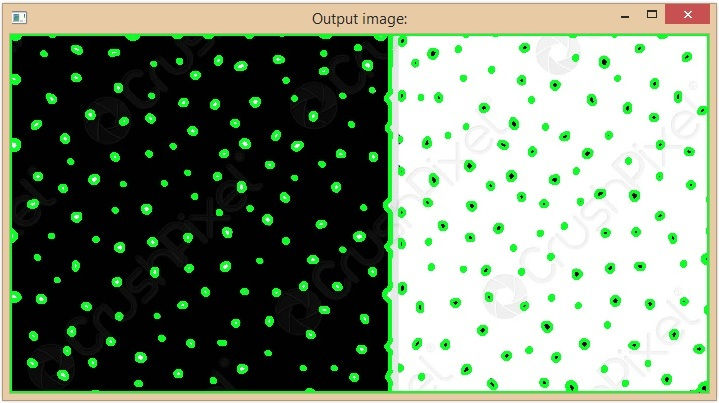## Using SimpleBlobDetector

In OpenCV, SimpleBlobDetector is a class that is used to extract the blobs from an image. The cv2.SimpleBlobDetector_create() method creates a detector to detect the blob points on the given image based on the SimpleBlobDetector algorithm.

### Example

In this example, we will use the cv2.SimpleBlobDetector_create() method to detect the black dots on a white background image.

import cv2
import numpy as np;

# create the detector with default parameters.
detector = cv2.SimpleBlobDetector_create()

# Detect dots.
keypoints = detector.detect(im)

print("Black Dots Count is:",len(keypoints))

# Draw detected blobs as red circles.
im_with_keypoints = cv2.drawKeypoints(im, keypoints, np.array([]), (0,0,250), cv2.DRAW_MATCHES_FLAGS_DRAW_RICH_KEYPOINTS)

cv2.imshow("Output image:", im_with_keypoints)
cv2.waitKey(0)


### Output

Black Dots Count is: 18


In the above code the cv2.DRAW_MATCHES_FLAGS_DRAW_RICH_KEYPOINTS is used ensures that the size of the circle corresponds to the size of blob. And in the output we can see that the total number of black dots are 49 in the given image.

### Input Image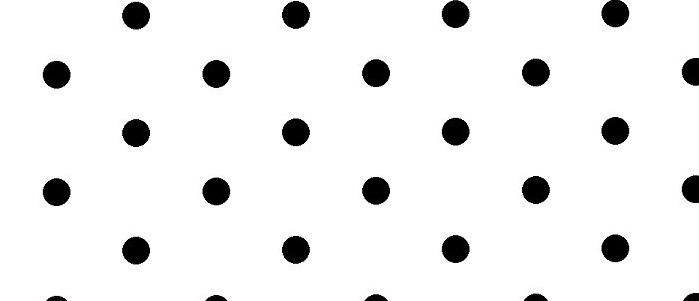### Output Image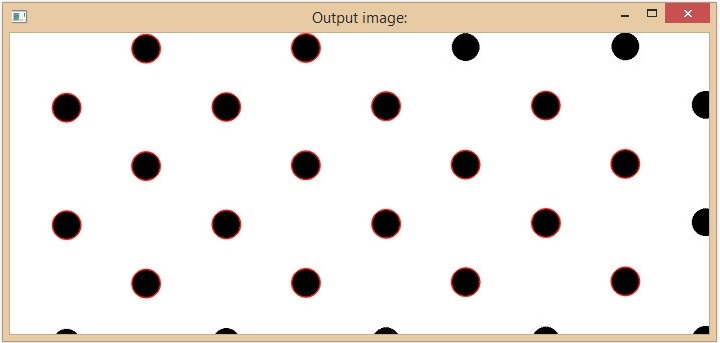Updated on: 30-May-2023

989 Views